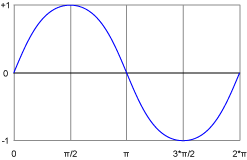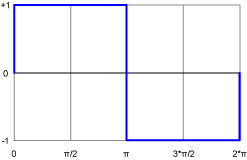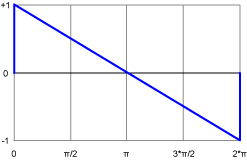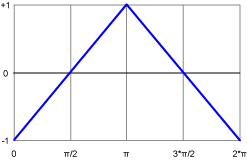# Sound Synthesis Part 2: Waveforms

In the previous part of this series you learned a lot about the mother of all periodic waveforms, the sine wave and how to create a simple tone generator that sounds the note A using AS3.

Yesterdays and todays synthesizers offer a variety of different sound tone generators called oscillators. What it actually sounds like among other factors depends on the waveform it generates. As of yet you know what a sine wave sounds like but what else is there?
There are three other common periodic waveforms, each unique in sound.

Square

To refresh your memory, the sine wave sweeps between -1 and +1 periodically or more generally between -amplitude and +amplitude in one cycle. One cycle goes from 0 to 2 * π.
The number of cycles it completes within one second make up the fundamental frequency of the sound.One sine wave cycle

In comparison, one cycle of a square wave looks like this:One square wave cycle

As you can see the difference is kinda obvious. The sound as well!
Let’s hear our note A again, this time generated using a square wave.

But how can we construct this waveform?
Let’s take a look at the code for our sinewave generator we developed in part 1 of this series:

Phase tells us at what position of the waveform we currently are and is a value between 0 and 2 * π. If it exceeds 2 * π we finished a cycle and can jump back to the beginning.
If we take a closer look at the square wave above, we notice that between 0 and π, the amplitude stays at +1 while between π and 2 * π the amplitude suddenly goes to -1 for the rest of the cycle. That shouldn’t be too hard to put into an algorithm.
In fact, it’s as easy as 1 2 3!
Pseudo code:
If phase is smaller than π then amplitude=1
If phase is greater than π then amplitude=-1

Transfered to AS3:

That’s it! The remaining two waveforms are just as easy!

Sawtooth

Again, we should have a look at a visual representation first.One sawtooth wave cycle

Can you imagine what it sounds like? If not, listen to this example:

Simply by looking at the graph, we can derive an algorithm. The amplitude goes from +1 to -1 gradually from 0 to 2 * π. If we remember phase being some kind of pointer, we can come up with:

Ready for the last waveform? 🙂

Triangle

I know you’re surprised now. A triangle waveform is shaped kinda like a triangle!One triangle wave cycle

Like a sine wave it’s very pure in sound and can be used as a basis for a flute for example.

By looking at the graph we can see that it’s amplitude rises gradually from -1 to -1 within π and descents back to -1 at 2 * π.
One way to express this as an alogrithm would be:

Summing up

Now that we know more about the common periodic waveforms sine, square, sawtooth and triangle let’s revise our tonegenerator from part 1 of the series. We want to incorporate the missing 3 oscillators!
Here’s the complete code first:

Again, this is pretty straightforward.

Line 15
We set up a new variable called oscillator, which makes it possible for us to select our desired oscillator. Possible values are sine, square, sawtooth and triangle.

Lines 39-53
Based on the value of oscillator, we pick one of the algorithms we’ve worked out in this tutorial.